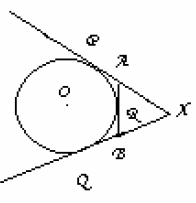## Prove that xa+ar=xb+br of circle, Mathematics

Assignment Help:

In figure, XP and XQ are tangents from X to the circle with centre O. R is a point on the circle. Prove that XA+AR=XB+BRAns:    Since the length of tangents from external point to a circle are equal

XP = XQ PA = RA BQ = BR

XP = XQ

⇒XA + PA = XB + BQ

⇒XA + AR = XB + BR (Θ PA = AR & BQ = BR)

Hence proved

#### Surface area- applications of integrals, Surface Area- Applications of inte...

Surface Area- Applications of integrals In this part we are going to look again at solids of revolution. We very firstly looked at them back in Calculus I while we found the

#### Maximum and minimum values, Find all the local maximum and minimum values a...

Find all the local maximum and minimum values and saddle points of the function f(x, y) = x 2 - xy + y 2 + 9x - 6y + 10

#### Differential equation, solve: 4ydx+xdy=0

solve: 4ydx+xdy=0

#### Example of the invisible effort, Imagine a time in history when the number ...

Imagine a time in history when the number system had not yet evolved a farmer needed to keep track of his cattle. What would he do to figure out whether his entire rattle returned

#### Explain factor by grouping, Explain Factor by Grouping ? Factoring by g...

Explain Factor by Grouping ? Factoring by grouping is often a good way to factor polynomials of 4 terms or more. (Sometimes it isn't. It doesn't always work. But it's worth try

#### Free Assignment Test Online, Well, my uncle want me to tutor him in mathema...

Well, my uncle want me to tutor him in mathematics. But, the problem is I don''t know what he already knows about math. It for his Compass Test when he go back to school in the spr

#### Transition matrix, example #Minimum 100 words accepted#

example #Minimum 100 words accepted#

#### Iti, Gm signal is better than am signal becuase

Gm signal is better than am signal becuase

#### Find out the area under the parametric curve, Find out the area under the p...

Find out the area under the parametric curve given by the following parametric equations.  x = 6 (θ - sin θ) y = 6 (1 - cos θ) 0 ≤ θ ≤ 2Π Solution Firstly, notice th